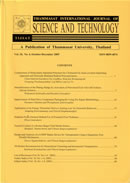THAMMASAT INTERNATIONAL JOURNAL OF SCIENCE & TECHNOLOGY

Volume 25, No. 01, Month JANUARY, Year 2020, Pages 85 - 94

An efficient computation approach for abel’s integral equations of the second kind

Rajendra K. Bairwa, Ajay Kumar, Devendra Kumar

Abstract

This paper presents the application of a new powerful method named as q-homotopy analysis transform method (q-HATM). The q-HATM is a combination of the q-homotopy analysis scheme and the Laplace transform approach and more general than other existing techniques. Abel’s integral equation of the second kind has been solved by using this method. We solve some examples and plot the graphs. The numerical solutions are shown in the form of graphs.

Keywords

Abel’s integral equation of second kind, Laplace transform, q-homotopy analysis transform method

THAMMASAT INTERNATIONAL JOURNAL OF SCIENCE & TECHNOLOGY